# Quiz 8: Underwriting and Financing Residential Properties

Annual Percentage Return Mr. X is a local resident of the USA. He made a fixed rate loan of amount \$70,000 for 25 year at 9% annual return. Payment as Installment should be paid on the monthly basis. The borrower/buyer should pay to Mr. X, \$1,500 as discount point. (a) Calculation of Annual percentage rate (APR) is provided in Excel sheet.Rows B2 to Row B7 provide the information about the loan and screen shot is provides below: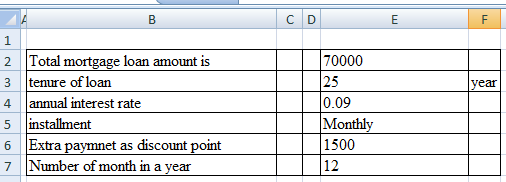Under the calculation box net present value of Mortgage loan is calculated by the actual loan minus the prepaid expenses. Cell (E12) shows the value of net present value of loan. Total number of period of installment is shows in cell (E13) which is calculated by multiplication of number of year and number of payment in a year. In cell (E16) value of installment per month is calculated by following formula: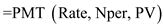Where, Rate is 9% Nper is 300 PV is \$70,000 Use the following screenshot to calculate the PMT: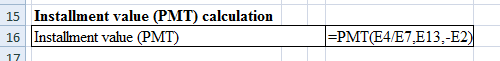In cell (E16) value of PMT is calculated as \$587.44. Based on PMT value of APR is calculated in Cell E(19) which is calculated by following formula: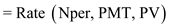Nper is 300 PMT is \$587.44 PV is net present value of loan that is \$68,500 Use the following screenshot to calculate the RATE: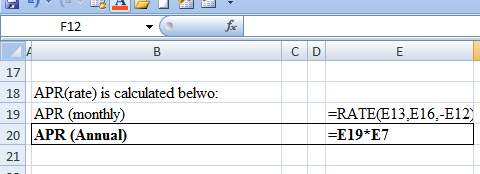From the calculation it is fount that APR monthly is 0.77% which shows in Cell (E19). Based on value of monthly APR, annual APR is calculated in cell (E20). Thus APR for the loan is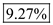(b) Actual value of return may differ from the annual percentage return because of following reasons: 1. In the APR calculation it is assume that prepaid expenses is paid by buyer/borrower is at the time of loan disbursement but in actual scenario prepaid expenses may pay later or before the loan disbursement. 2. The lender has some other operating expenses in disbursement of loan which is not considered in the APR calculation. 3. Lender's salary is not considered in APR calculation.
The APR stands for the Annual Percentage Rate. It indicates the stated rate of interest on the loan/investment. In order to determine which loan/investment offering is beneficial the APR should be known. The loan with lower APR is preferable. Federal lending truth (FTL) is a legislation which is intended to protect the consumer interest. Under the FTL lender should disclose all the financial information in loan agreement in comparable manner. So, that the consumer can compare the loan amount and interest rate among different loan provider. Like the RESPA disclosure which is based on estimates, under the FTL disclosure the Annual percentage rate (APR) is accurately discloses under the FTL Disclosure. The calculation of Annual percentage rate by the lender may be rounded up to nearest one quarter of a percentage. After rounding the Annual percentage rate adjusted in such a way that it must be closer to the one eighth of the percentage Annual percentage rate that is calculated based on FTL guidance. If the interest rate and so the Annual percentage rate change from the date of loan application to closing of loan the lender requires to make some additional disclosure. a. Compute the APR of the worst case when the borrower could take decision of pre-closure of the loan using the spreadsheet. Enter values and formulas in the spreadsheet as shown in the image below.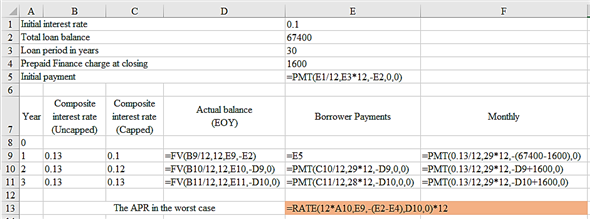The obtained result is provided below.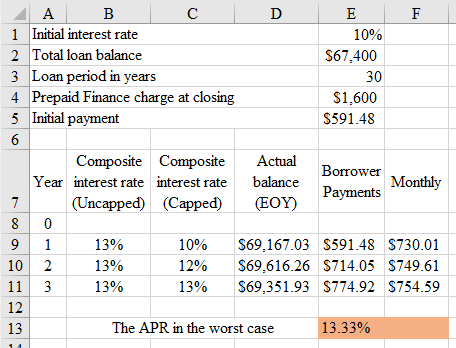Thus, the APR to the borrower is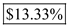. b. In the case of Adjustable Rate Mortgage (ARM) it difficult to determine the true APR. The APR truly help the borrowers to know the effective cost of borrowing and to make comparison among the available alternatives. The problem is that the APR does not reflect the true cost of borrowing in the case of ARM the composite interest rate remains constant throughout the life of the company but it is not the actual interest rate that the borrower is paying.